Effect Of Capillary Rise Of Water On Effective Stress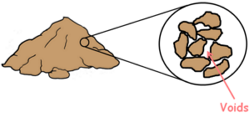A soil mass is composed of small solid particles which we call the soil grains. These soil grains when depositing in a soil mass arranges themselves in a way that some amount of empty space is enclosed between them. We call these spaces voids.

These soil particles in a soil mass are burdened by the weight of everything above them which includes weight of all the soil particles above them, weight of water and weight of any structure if present above; and because of that these particles experience stress which we call total stress.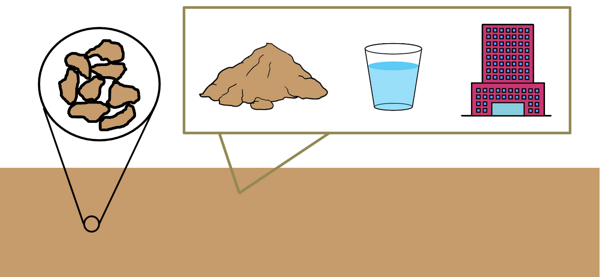But if we put this soil mass in water then because of buoyancy force the net total downward force that is acting on the soil particles is reduced. Consequently the stress on particles is reduced. This reduced stress is called effective stress.

The stress exerted by the water present in the soil pores is called the pore water pressure.

We can write expression for effective stress as this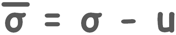Effective stress is different at different depths below ground surface because total stress and pore water pressure changes at different depth.

We have already discussed the effect of variation of water table on the effective stress. Read that article here.

We know that stress at any point or plane under the soil can be given as force on that plane divided by the area of the plane.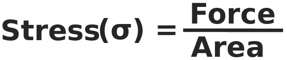Force on that plane is the weight of soil above the plane and that is unit weight of soil multiplied by its volume. Volume is area multiplied by the height of the soil mass.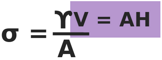This can be simplified to this:

σ  = γH

So we can directly write the stress under any material as unit weight of the material multiplied by the height of the material above that point.

We know that water molecules travel in upward direction in the soil even against the gravitational forces because of a phenomenon called the capillarity or capillary effect.

Capillary effect is the ability of a liquid to flow in narrow spaces without the assistance of external forces. This risen water is called capillary water.

We will try to calculate the effective stress in soil at different depth when there is a capillary rise in the soil.

So let us consider a soil mass of bulk unit weight γ and assume the water table lies at some depth H1 below surface.

Because of the water table soil becomes completely saturated and unit weight of soil becomes γsat. The water below water table is in compression because of the weight of water itself and the pore water pressure is positive that is above atmospheric pressure.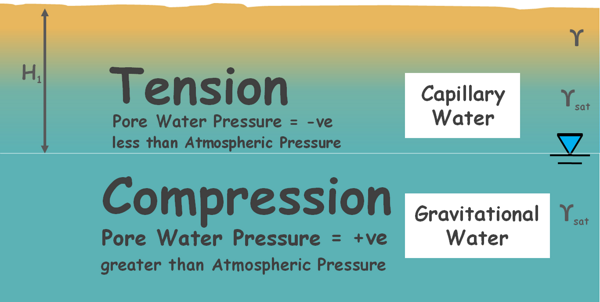Soil above the water table becomes saturated because of capillary rise of water. So unit weight of this soil also becomes γsat. In case of capillary rise, water above the water table is in tension because it is pulled up by inter-molecular forces and the pore water pressure in this region is negative that is below atmospheric pressure.

Let us consider the capillary water has risen up to the surface and the soil becomes fully saturated up to the surface. We will try to determine the effective stress at different depths.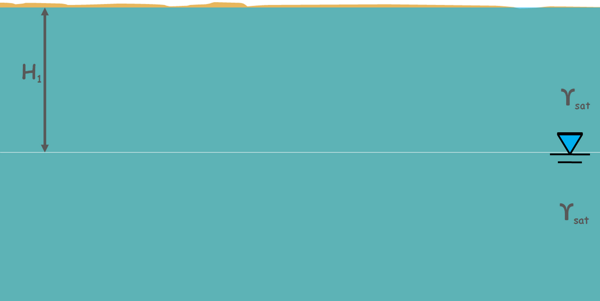Let’s begin with the surface. The total stress at the surface is zero because no load is present above this plane.

σ = 0

No water is present above this plane, but capillary water is present here and it is in tension. So pore water pressure is not zero at the surface. It is unit weight of water γw multiplied by height up to which the capillary water has risen that is H1 and it is negative as it is below atmospheric pressure.

u = -γwH1

So effective stress at the surface of the soil is given as total stress minus pore water pressure.

σ’ = σ - u

σ’ = 0 - (-γwH1)

And we can write it as this.

σ’ = γwH1

If the capillary water is absent in the soil then at the surface pore water pressure would be zero and so would be effective stress.

Now analyze the stresses below the ground surface at some plane in capillary saturation zone.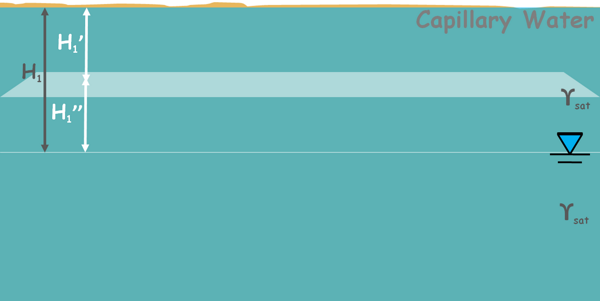The total stress on this plane is because of this saturated soil above gamma sat multiplied by height of the soil above this plane say H1’.

σ = γsatH1

Pore water pressure at this plane is negative because of capillary water present and is gamma w multiplied by the height of the capillary rise up to this plane say H1’’.

u = -γwH1’’

We can also write this height as H1 minus H1

H1’’ =H1 - H1

So pore water pressure can be written as this:

u = -γw(H1- H1’)

We should note that water present above this plane does not cause to increase pore water pressure at this plane because this water is also capillary water and is in tension. It cannot compress the water below.

So effective stress at this surface can be written as this:

σ’ = σ - u

σ’ = γsatH1’ - (-γw(H1- H1’))

By solving we can write it is this.

σ’ = γsatH1’ - γwH1- γwH1

σ’ = (γsat - γw)H1’ + γwH1

and this quantity can be written as submerged unit weight of soil.

σ’ = γ’H1’ + γwH1

Now let’s analyze the stresses at the plane present at water table.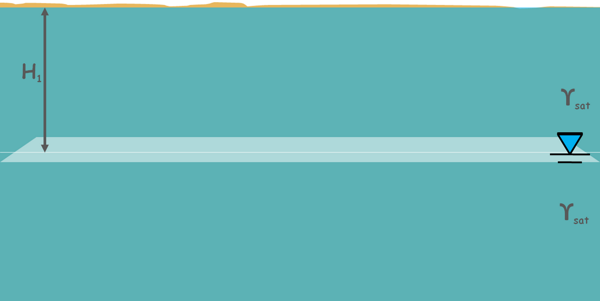The total stress on this plane because of the saturated soil above gamma sat H1.

σ = γsatH1

Pore water pressure at this plane is zero because water on the water table remains at atmospheric pressure and water is neither in tension nor in compression.

u = 0

So effective stress will be equal to total stress:

σ’ = σ - u

σ’ = γsatH1

Now move down the water table and analyze the stresses at a plane that is say at H2 depth below water table.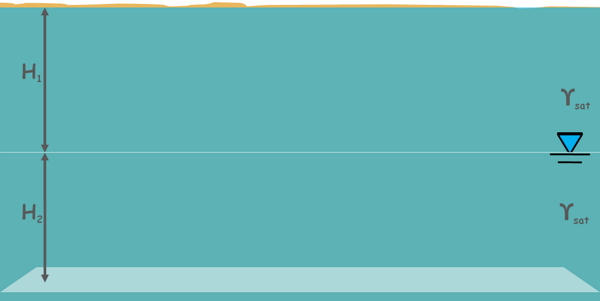The total stress on this plane is because of the saturated soil above the water table of height H1, gamma sat H1 plus stress because of saturated soil below the water table of height H2, gamma sat H2.

σ = γsatH1 + γsatH2

Pore water pressure at this plane is compressive and is because of water below water table. Water above the water table has no effect under the water table.

So pore water pressure is positive gamma w H2.

u = γwH2

Hence effective stress at this surface can be written as this:

σ’ = σ - u

σ’ = γsatH1 + γsatH2 - γwH2

And we can write this quantity as submerged unit weight of soil.

σ’ = γ’H1 + γ’H2

Now let us take another case when capillary rise saturates the soil only up to some height above the water table and soil saturation does not reach up to the surface. Saturated part of the soil has unit weight gamma sat and not-saturated perhaps wet soil has bulk unit weight gamma.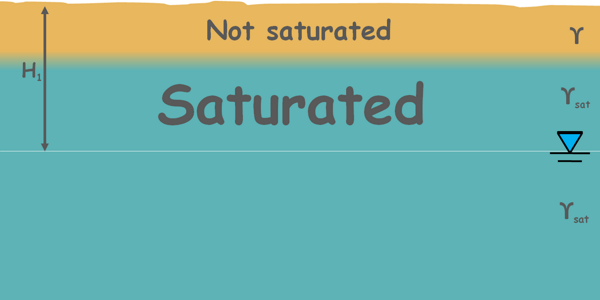Let’s begin the analysis with the soil surface again.

The total stress at the surface is zero.

σ = 0

pore water pressure is also zero as no water is present above the surface and no capillary water rose to the surface.

u = 0

So effective stress at the surface is also zero.

σ’ = σ - u

σ’ = 0

Now let’s analyze stresses at the plane, up to which the capillary water has saturated the soil.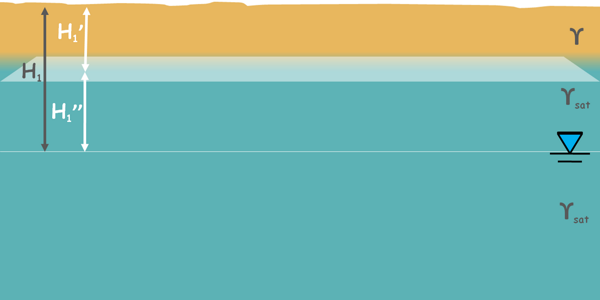The total stress on this plane is because of the soil above this plane, bulk unit weight gamma and height of the soil above this plane say H1’.

σ = γH1

Pore water pressure at this plane is negative gamma w and height of this plane above water table say H1’’.

u = -γwH1’’

So effective stress at this surface can be written as this:

σ’ = σ - u

σ’ = γH1’ - (-γwH1’’)

By solving we can write it is this.

σ’ = γH1’ + γwH1’’

Now let’s analyze the stresses on a plane at water table.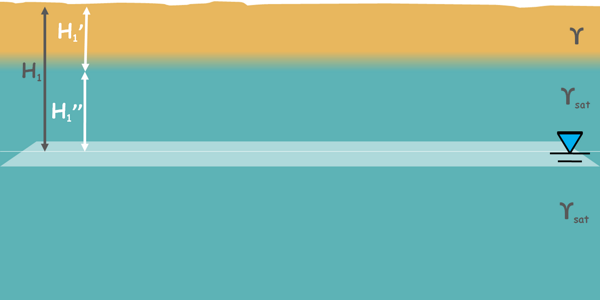The total stress on this plane because of wet soil gamma H1’ plus stress because of capillary saturated soil gamma sat H1’’.

σ = γH1’ + γsatH1’’

Pore water pressure at this plane is zero as water is at water table and at atmospheric pressure.

u = 0

So effective stress will be equal to total stress:

σ’ = σ - u

σ’ = γH1’ + γsatH1’’

Now move below the water table and analyze the stresses at this plane which say at H2 depth.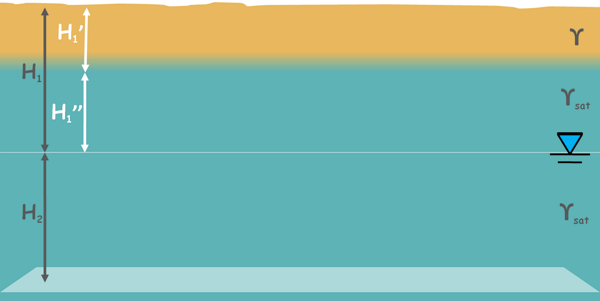The total stress on this plane is because of wet soil gamma H1’ plus because of capillary saturated soil above the water table gamma sat H1’’ plus because of saturated soil below the water table gamma sat H2.

σ = γH1’ + γsatH1’’ + γsatH2

Pore water pressure at this plane is in compression and is because of water that is below water table gamma w H2.

u = γwH2

So effective stress at this surface can be written as this:

σ’ = σ - u

σ’ = γH1’ + γsatH1’’ + γsatH2 - γwH2

We can also write this as submerged unit weight of soil.

σ’ = γH1’ + γsatH1’’ + γ’H2

Tags : capillarity

Published on :2020-07-29